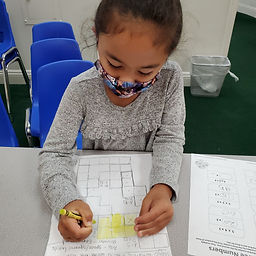Ms. Raejean

Target 1​

Lesson Type:

New

Number Operation

:

Computation

Multiply within 100.

1:

Understand that the same process is utilized to multiply 3 or more whole numbers that is used to multiply only two numbers (i.e., 2x3x4 = 2 groups of 3 in 6, and 4 groups of 6 is 24).

3rd

Vocabulary:

Factors, Product, Commutative Property, Arrays (rectangular arrangement in rows & columns)

Activities:

• We reviewed commutative property. Equations have the same factors (numbers) being multiplied, the same product (answer), but the order in which the numbers are multiplied are switched. Ex. 4 x 6 = 6 x 4
• Students selected 3 cards to make a multiplication equation. We discussed a strategy for multiplying the 3 numbers. Multiply the first and second factors (numbers), then multiply the product (answer) by the third factor. We also talked about switching the order of the 3 numbers for easier computation. Ex. 3 x 6 x 2 = 18 x 2 vs. 6 x 2 x 3 = 12 x 3
• Students used strategies (ex. used counters/made arrays, skip counted, repeated addition) to compute. Students practiced and applied what they learned in their workbooks (given arrays) and on a take-home worksheet.Home Exploration

Guiding Questions:Absent Students:

Target 2

:

1:

Solve pentomino puzzles by recognizing which spaces in the puzzle the given 12 pieces can and cannot fit.

2:

Recognize and apply reflections (flips), translations (slides), and rotations (turns).

3:

Identify the relationship between the 12 pentomino figures and their area and perimeter.

3rd

Vocabulary:

Pentomino, Area (squares inside), Perimeter (edges around)

Activities:

• Students demonstrated their spatial abilities as they worked to fill a 10 by 6 grid with the given 12 pentominoes. We reviewed slides, flips, and turns as students solved the problem of filling the 10 by 6 grid.
• We learned about area (squares inside) and perimeter (edges around). Students were challenged to find the "magic pentomino," which is the one that is unlike the rest. The "magic penomino" has a perimeter of 10 vs 12.Home Exploration

Guiding Questions:Target 3

:

Vocabulary:

Activities:Home Exploration